Since you can describe a point in the plane with two numbers, why would you choose to use three numbers? Why would you ever want to use a coordinate system with more coordinates than necessary?

## Barycentric coordinates

One way to indicate the location of a point inside a triangle is to give the distance to each of the vertices. These three distances are called barycentric coordinates. Why would you use three numbers when two would do?

Barycentric coordinates make some things much simpler. For example, the coordinates of the three vertices are (1, 0, 0), (0, 1, 0), and (0, 0, 1) for any triangle. The points inside are written as convex combinations of the vertices. The coordinates of the center of mass, the barycenter, are (1/3, 1/3, 1/3). The vertices are treated symmetrically, even if the triangle is far from symmetric.

Barycentric coordinates are useful in applications, such as computer graphics and finite element analysis, because they are relative coordinates. When a triangle moves or is rescaled, you only need to keep track of where the vertices went; the coordinates of the points inside relative to the vertices haven’t changed.

This can be generalized to more dimensions. For example, you could describe a point in a tetrahedron with four coordinates, more in higher dimensions you could describe a point in an n-simplex by the convex combination coefficients of the n vertices.

Barycentric coordinates are related to Dirichlet probability distributions. When you have n probabilities that sum to 1, you’ve got n-1 degrees of freedom. But it often simplifies things to work with n variables. As with the discussion of triangles above, the extra variable makes expressions more symmetric.

## Quaternions and rotations

A point in three dimensional space can be described with three numbers, but it’s often useful to think of the usual three coordinates as the vector part of a quaternion, a set of four numbers.

Suppose you have a point

a = (x, y, z)

and you want to rotate it by an angle θ around an axis given by a unit vector

b = (u, v, w).

You can compute the rotation by associating the point a with the quaternion

p = (0, x, y, z)

and the axis b with the quaternion

q = (cos(θ/2), sin(θ/2) u, sin(θ/2) v, sin(θ/2) w)

The image of a is then given by the quaternion

q p q-1.

This quaternion will have zero real part, and so the Euclidean coordinates are given by the vector part, the last three components.

Of course the product above is a quaternion product, which is not commutative. That’s why the q and the q-1 don’t cancel out.

Using quaternions for rotations has several advantages over using rotation matrices. First, the quaternion representation is more compact, describing a rotation with four real numbers rather than nine. Second, the quaternion calculation can be better behaved numerically. But most importantly, the quaternion approach avoids gimbal lock, a sort of singularity in representing rotations.

## Projective planes

In applications of algebra, such as elliptic curve cryptography, you often need to add “points at infinity” to make things work out. To formalize this, you add an extra coordinate. So while an elliptic curve is usually presented as an equation such as

y² = x³ + ax + b,

it’s more formally an equation in three variables

y²z = x³ + axz² + bz³.

Points in the projective plane have coordinates (x, y, z) where points are considered equivalent if they differ by a non-zero multiple, i.e. (x, y, z) is considered the same point as (λx, λy, λz) for any non-zero λ.

You can often ignore the z, choosing λ so that the z coordinate is 1. But when you need to work with the point at infinity in a uniform way, you bring out the full coordinates. Now the “point at infinity” is not some mysterious entity, but simply the point (0, 1, 0).

## Common themes

Projective coordinates, like barycentric coordinates, introduce symmetry. With the addition of an extra coordinate, the three coordinates all behave similarly, with no reason to distinguish any coordinate as special. And as with quanternion rotations, projective coordinates make singularities go away, which is consequence of symmetry.

# Quaternion reference in the Vulgate

To contemporary ears “quaternion” refers to a number system discovered in the 19h century, but there were a couple precedents. Both refer to something related to a group of four things, but there is no relation to mathematical quaternions other than that they have four dimensions.

I’ve written before about Milton’s use of the word in Paradise Lost. Milton is alluding to the four elements of antiquity: air, earth, fire, and water.

I recently found out the word quaternion appears in the Latin Vulgate as well. Acts 12:4 records that Herod had Peter guarded by four squads of four soldiers each, “quattuor quaternionibus militum.”

Update: Thanks to Phil for pointing out in the comments that quaternion appears in the King James as well, “four quaternions of soldiers.”

# Redoing division algebra graphs with angular distance

The blog post that kicked off the recent series of posts looked at how far apart xy and yx are for quaternions. There I used the Euclidean distance, i.e. || xy – yx ||. This time I’ll look at the angle between xy and yx, and I’ll make some analogous graphs for octonions.

For vectors x and y in three dimensions, the dot product satisfieswhere θ is the angle between the two vectors. In higher dimensions, we can turn this theorem around and use it as the definition of the angle between two vectors based on their dot product.

(The plots below are jagged because they’re based on random sampling.)

Here’s the Euclidean distance between xy and yx for quaternions from the earlier post: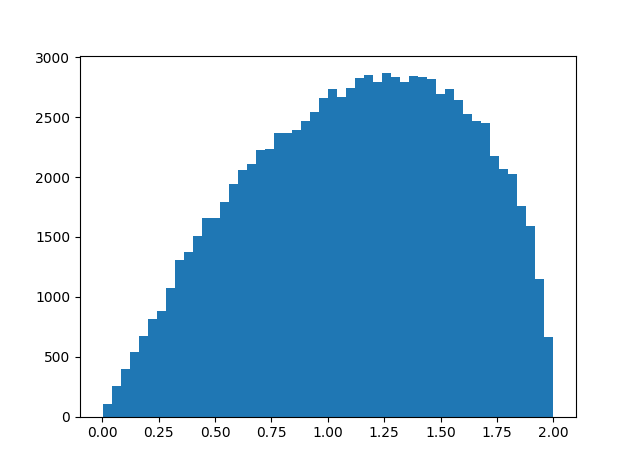And here’s the corresponding angular distance:The range has changed from [0, 2] to [o, π], and the distribution now shifts left instead of right.

Here’s a similar graph, looking at the angular distance between xy and yx for octonions, something I haven’t plotted before in Euclidean distance.This graph is more symmetric, which we might expect: since octonions have less algebraic structure than quaternions, we might expect the relationship between xy and yz to behave more erratically, and for the plot to look more like a normal distribution.

Finally, let’s revisit the distance between (xy)z and x(yz) for octonions. Here is the distribution of the Euclidean distance from a previous post: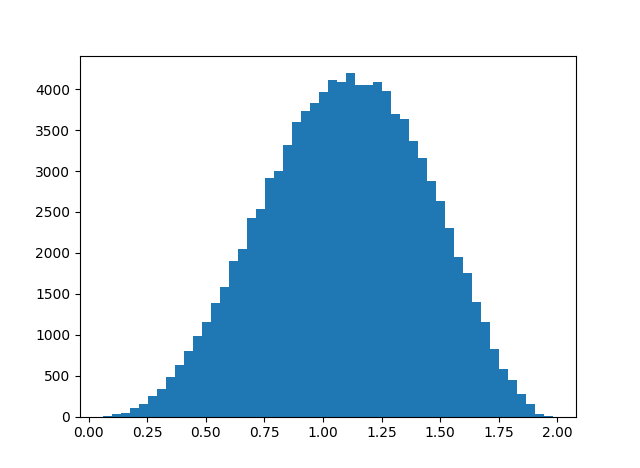And here is the corresponding histogram based on angular distance.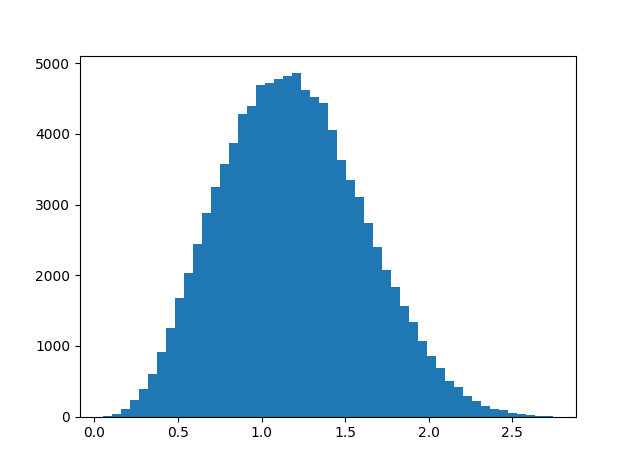These plots are based on uniform random samples of quaternions and octonions of length 1, i.e. points from the unit spheres in 4 and 8 dimensions respectively. Quaternions and octonions have the property that the product of unit length vectors is another unit length vector, and the angle between to unit vectors is the inverse cosine of their dot product.

I thought that sedenions also had this norm property, that the product of unit length vectors has unit length. Apparently not, as I discovered by trying to take the inverse cosine of a number larger than 1. So what is the distribution of lengths that come from multiplying two sedenions of length 1? Apparently the mean is near 1, and here’s a histogram.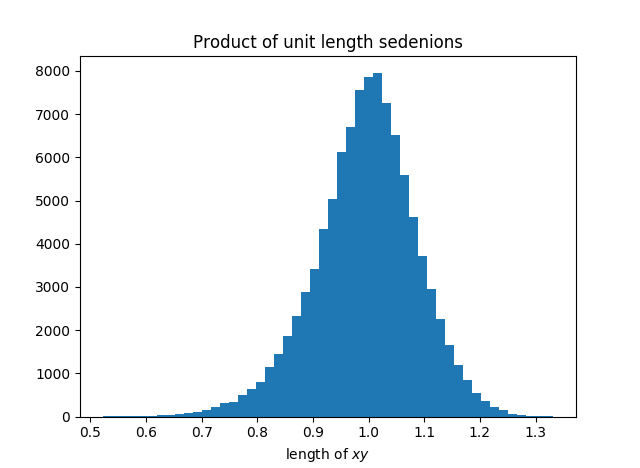# Refactored code for division algebras

An earlier post included code for multiplying quaternions, octonions, and sedenions. The code was a little clunky, so I refactor it here.

    def conj(x):
xstar = -x
xstar *= -1
return xstar

def CayleyDickson(x, y):
n = len(x)

if n == 1:
return x*y

m = n // 2

a, b = x[:m], x[m:]
c, d = y[:m], y[m:]
z = np.zeros(n)
z[:m] = CayleyDickson(a, c) - CayleyDickson(conj(d), b)
z[m:] = CayleyDickson(d, a) + CayleyDickson(b, conj(c))
return z


The CayleyDickson function implements the Cayley-Dickson construction and can be used to multiply real, complex, quaternion, and octonion numbers. In fact, it can be used to implement multiplication in any real vector space of dimension 2n. The numeric types listed above correspond to n = 0, 1, 2, and 3. These are the only normed division algebras over the reals.

When n = 4 we get the sedenions, which are not a division algebra because they contain zero divisors, and the code can be used for any larger value of n as well. As noted before, the algebraic properties degrade as n increases, though I don’t think they get any worse after n = 4.

If you wanted to make the code more robust, you could add code to verify that the arguments x and y have the same length, and that their common length is a power of 2. (You could just check that the length is either 1 or even; if it’s not a power of 2 the recursion will eventually produce an odd argument.)

# How close is octonion multiplication to being associative?

Quaternion multiplication is associative but not commutative. An earlier post looked at the average size of the commutator xy – yx as a measure of how far quaternion multiplication is from being commutative.

This post looks at an analogous question for octonions. Octonion multiplication is neither commutative nor associative. So in this post we look at the associator of three octonions (xy)z – x(yz) as a measure of how far octonion multiplication is from being associative.

(A post from yesterday looked at how close octonion multiplication comes to being associative in an algebraic sense, looking at weak forms of associativity. This post looks at how close multiplication comes to being associative in an analytical sense, looking at norm distances rather than algebraic identities.)

We will use a simulation to look at the average norm of the octonion associator over the octionions of unit length, analogous to the earlier post that looked at the commutator of the quaternions. We developed code for octonion multiplication in the previous post and will reuse that code here. We also developed code for generating random unit-length octonions in the same post.

Here’s code to find the average norm of the associator and plot a histogram of its values.


import matplotlib.pyplot as plt

# omult is octonion multiplication.
# See previous post.
def associator(x, y, z):
return omult(omult(x, y), z) -
omult(x, omult(y, z))

N = 100000
s = 0
h = np.zeros(N)
for n in range(N):
x = random_unit_octonion()
y = random_unit_octonion()
z = random_unit_octonion()
t = norm(associator(x, y, z))
s += t
h[n] = t

print(s/N)
plt.hist(h, bins=50)
plt.show()


This gives an average of 1.095 and the histogram below.Update: Greg Egan calculated the exact mean value for the norm of the associator to be 147456/(42875 π) ≈ 1.0947336. Here are the details.

# How far is xy from yx on average for quaternions?

Given two quaternions x and y, the product xy might equal the product yx, but in general the two results are different.

How different are xy and yx on average? That is, if you selected quaternions x and y at random, how big would you expect the difference xy – yx to be? Since this difference would increase proportionately if you increased the length of x or y, we can just consider quaternions of norm 1. In other words, we’re looking at the size of xy – yx relative to the size of xy.

Here’s simulation code to explore our question.

    import numpy as np

def random_unit_quaternion():
x = np.random.normal(size=4)
return x / np.linalg.norm(x)

def mult(x, y):
return np.array([
x*y - x*y - x*y - x*y,
x*y + x*y + x*y - x*y,
x*y - x*y + x*y + x*y,
x*y + x*y - x*y + x*y
])

N = 10000
s = 0
for _ in range(N):
x = random_unit_quaternion()
y = random_unit_quaternion()
s += np.linalg.norm(mult(x, y) - mult(y, x))

print(s/N)


In this code x and y have unit length, and so xy and yx also have unit length. Geometrically, x, y, xy, and yx are points on the unit sphere in four dimensions.

When I ran the simulation above, I got a result of 1.13, meaning that on average xy and yx are further from each other than they are from the origin.

To see more than the average, here’s a histogram of ||xyyx|| with N above increased to 100,000.I imagine you could work out the distribution exactly, though it was quicker and easier to write a simulation. We know the distribution lives on the interval [0, 2] because xy and yx are points on the unit sphere. Looks like the distribution is skewed toward its maximum value, and so xy and yz are more likely to be nearly antipodal than nearly equal.

Update: Greg Egan worked out the exact mean and distribution.

# Random walk on quaternions

The previous post was a riff on a tweet asking what you’d get if you extracted all the i‘s, j‘s, and k‘s from Finnegans Wake and multiplied them as quaternions. This post is a probabilistic variation on the previous one.

If you randomly select a piece of English prose, extract the i‘s, j‘s, and k‘s, and multiply them together as quaternions, what are you likely to get?

The probability that a letter in this sequence is an i is about 91.5%. There’s a 6.5% chance it’s a k, and a 2% chance it’s a j. (Derived from here.) We’ll assume the probabilities of each letter appearing next are independent.

You could think of the process multiplying all the i‘s, j‘s, and k‘s together as a random walk on the unit quaternions, an example of a Markov chain. Start at 1. At each step, multiply your current state by i with probability 0.915, by j with probability 0.02, and by k with probability 0.065.

After the first step, you’re most likely at i. You could be at j or k, but nowhere else. After the second step, you’re most likely at -1, though you could be anywhere except at 1. For the first several steps you’re much more likely to be at some places than others. But after 50 steps, you’re about equally likely to be at any of the eight possible values.

Related post: How far are quaternions from being commutative?

# Wolfram Alpha, Finnegans Wake, and QuaternionsI stumbled on a Twitter account yesterday called Wolfram|Alpha Can’t. It posts bizarre queries that Wolfram Alpha can’t answer. Here’s one that caught my eye.

Suppose you did extract all the i‘s, j‘s, and k‘s from James Joyce’s novel Finnegans Wake. How would you answer the question above?

You could initialize an accumulator to 1 and then march through the list, updating the accumulator by multiplying it by the next element. But is there a more efficient way?

Quaternion multiplication is not commutative, i.e. the order in which you multiply things matters. So it would not be enough to have a count of how many times each letter appears. Is there any sort of useful summary of the data short of carrying out the whole multiplication? In other words, could you scan the list while doing something other than quaternion multiplication, something faster to compute? Something analogous to sufficient statistics.

We’re carrying out multiplications in the group Q of unit quaternions, a group with eight elements: ±1, ±i, ±j, ±k. But the input to the question about Finnegans Wake only involves three of these elements. Could that be exploited for some slight efficiency?

How would you best implement quaternion multiplication? Of course the answer depends on your environment and what you mean by “best.”

Note that we don’t actually need to implement quaternion multiplication in general, though that would be sufficient. All we really need is multiplication in the group Q.

You could implement multiplication by a table lookup. You could use an 8 × 3 table; the left side of our multiplication could be anything in Q, but the right side can only be ij, or k. You could represent quaternions as a list of four numbers—coefficients of 1, ij, and k—and write rules for multiplying these. You could also represent quaternions as real 4 × 4 matrices or as complex 2 × 2 matrices.

If you have an interesting solution, please share it in a comment below. It could be interesting by any one of several criteria: fast, short, cryptic, amusing, etc.

Update: See follow up post, Random walk on quaternions

# Mathematical modeling in Milton

In Book VIII of Paradise Lost, the angel Raphael tells Adam what difficulties men will have with astronomy:

Hereafter, when they come to model heaven
And calculate the stars: how they will wield the
The mighty frame, how build, unbuild, contrive
To save appearances, how gird the sphere
With centric and eccentric scribbled o’er,
Cycle and epicycle, orb in orb.Related post Quaternions in Paradise Lost

Last night I checked a few books out from a library. One was Milton’s Paradise Lost and another was Kuipers’ Quaternions and Rotation Sequences. I didn’t expect any connection between these two books, but there is one.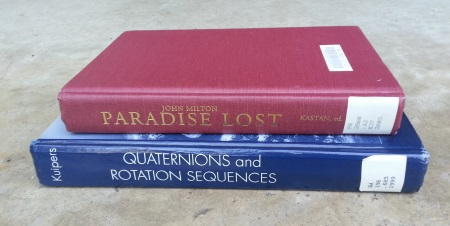The following lines from Book V of Paradise Lost, starting at line 180, are quoted in Kuipers’ book:

Air and ye elements, the eldest birth
Of nature’s womb, that in quaternion run
Perpetual circle, multiform, and mix
And nourish all things, let your ceaseless change
Vary to our great maker still new praise.

When I see quaternion I naturally think of Hamilton’s extension of the complex numbers, discovered in 1843. Paradise Lost, however, was published in 1667.

Milton uses quaternion to refer to the four elements of antiquity: air, earth, water, and fire. The last three are “the eldest birth of nature’s womb” because they are mentioned in Genesis before air is mentioned.